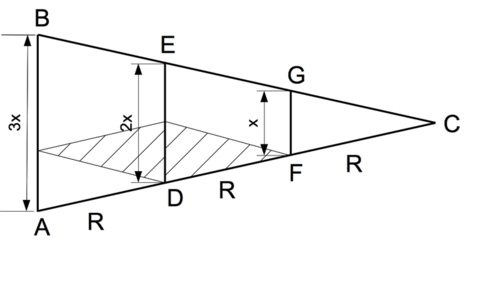•• Contributed by CK12
• CK12

In a lunar eclipse, if the width of the shadow of the Earth is twice the width of the Moon, then the width of the Earth itself is (very nearly) three times that of the Moon -- not twice, as one might perhaps think. Here is why:

The Sun is not a point of light but an extended source, with a disk covering a circular patch in the sky, about 0.5 degrees across. This makes the shadow of the Earth not a cylinder, stretching to infinity without narrowing down, but a cone, with an angle of 0.5 degrees across its apex C (drawing). AB is here the diameter of the Earth, and the directions AC and BC represents rays from opposite edges of the Sun's disk, rays whose directions differ by 0.5 degrees.Figure $$\PageIndex{1}$$: Illustration of a Lunar eclipse.

If $$x$$ is the diameter of the Moon and $$R$$ its distance, then according to Aristarchus, the width ED of the shadow at distance $$R$$ equals $$2x$$ (actually, $$2.5x$$ comes closer to the mark). We add to the drawing points H and K so that HA = KD = $$x$$.

The width of the Moon as seen from point H is KD = $$x$$, and since the Moon's size in the sky is about the same as the Sun's, the angle KHD (shaded) should also equal 0.5 degrees. We now extend the line AD = R a further distance R to point F. Then the two shaded triangles HKD and KFD are congruent (= same in size and shape) and have the same 0.5 angle as the angle at C. Indeed, one can prove now that the triangles GFC and AHD are also congruent to the two shaded ones.

It follows then that AC = $$3R$$, and from simple proportions (see drawing) $$AB\,=\,3x$$.